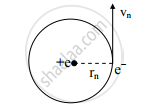HSC Science (Computer Science) 12th Board ExamMaharashtra State Board
Share

Derive an Expression for the Total Energy of Electron in ‘N' Th Bohr Orbit. - HSC Science (Computer Science) 12th Board Exam - Physics

ConceptMass-energy and Nuclear Binding Energy Nuclear Binding Energy

Question

Derive an expression for the total energy of electron in ‘n' th Bohr orbit. Hence show that energy of the electron is inversely proportional to the square of principal quantum number. Also define binding energy.

Solution

Expression for total energy of electron in the nth orbit (En)

i. Kinetic energy (K.E.):
Let, m = mass of electron
rn = radius of nth orbit of Bohr’s hydrogen atom
vn = velocity of electron
−e = charge of electron
+e = charge on the nucleusAccording to Bohr’s first postulate,

(mv_n^2)/r_n=1/(4piin_0)xxe^2/r_n^2

where, ε0 is permittivity of free space.

(mv_n^2)=1/(4piin_0)xxe^2/r_n      ................(1)

The revolving electron in the circular orbit has linear speed and hence it possesses kinetic energy.
It is given by,

K.E=1/2mv_n^2

∴K.E =1/2 1/(4piin_0)xxe^2/r_n  from equation (1)

∴K.E=e^2/(8piin_0r_n)   ............................(2)

ii. Potential energy (P.E.):
Potential energy of electron is given by, P.E = V(−e)
where, V = electric potential at any point due to charge on nucleus
− e = charge on electron.

P.E=1/(4piin_0)e/r_nxx(-e)

P.E=1/(4piin_0) xx -e^2/r_n

P.E=-e^2/(4piin_0r_n)  ........................ (3)

iii. Total energy (En):
The total energy of the electron in any orbit is its sum of P.E and K.E.
∴ T.E = K.E + P.E

=e^2/(8piin_0r_n) + -e^2/(4piin_0r_n)    [From equations (ii) and (iii)]

E_n=-1/(8piin_0) e^2/r_n

E_n=-e^2/(8piin_0r_n)           .................(4)

iv. But, r_n=((in_0h^2)/(pime^2))n^2

Substituting for rn in equation (iv),

E_n=-1/(8piin_0) e^2/(((in_0h^2)/(pime^2))n^2)

=-1/(8piin_0) (e^2pime^2)/(in_0h^2n^2)

=-((me^4)/(8in_0^2h^2))1/n^2 ..........(5)

This is required expression for energy of electron in nth orbit of Bohr’s hydrogen atom

v. The negative sign in equation (v) shows that the electron is bound to the nucleus by an attractive force and hence energy must be supplied to the electron in order to make it free from the influence of the nucleus.

vi. Now, as m, e, ε0, and h in equation (v) are constant,

(me^4)/(8in_0^2h^2)=constant

Using equation (v), we get,

E_n="constant"(1/n^2)

E_n prop 1/n^2

Hence, the total energy of electron in a Bohr’s orbit is inversely proportional to the square of the principal quantum number.

The amount of energy required to separate all the nucleons from the nucleus is called binding energy of the nucleus.

Is there an error in this question or solution?

APPEARS IN

2013-2014 (October) (with solutions)
Question 8.2 | 7.00 marks

Video TutorialsVIEW ALL 

Solution Derive an Expression for the Total Energy of Electron in ‘N' Th Bohr Orbit. Concept: Mass-energy and Nuclear Binding Energy - Nuclear Binding Energy.
S